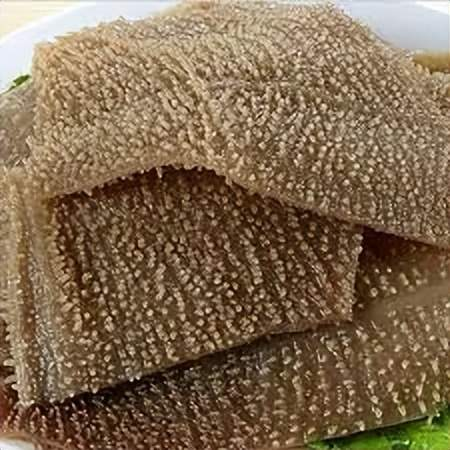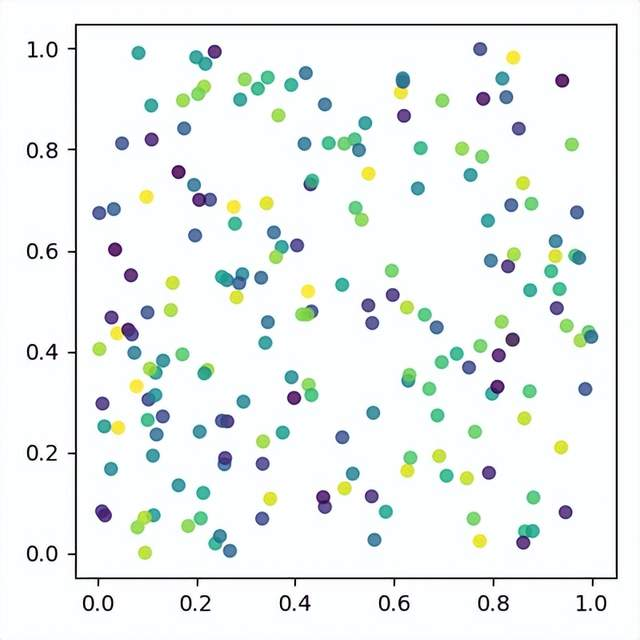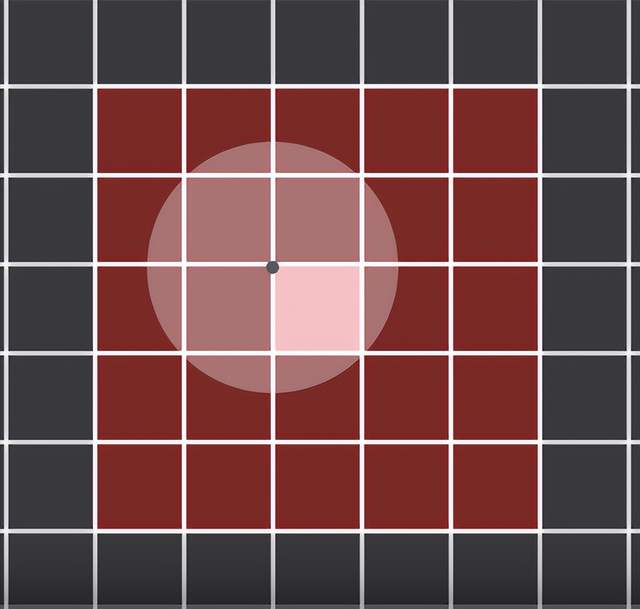# 太极图形60行代码实现经典论文，0.7秒搞定泊松盘采样，比Numpy实现快100倍# 采用Bridson算法实现``````ti.init(arch=ti.cpu)
``````

# 安装和导入Taichi

``````pip install taichi==1.0.3
``````

``````import taichi as ti
import taichi.math as tm``````

# 准备工作1. samples保存当前所有已经采样点的坐标，它的每个元素是一个二维坐标（x,y）。
2. grid[i, j]是一个整数，它存储的是第（i, j）个方格中采样点在数组samples中的下标。grid[i, j] = -1表示这个方格中没有采样点。

``````grid_n = 400
res = (grid_n, grid_n)
dx = 1 / res
inv_dx = res
desired_samples = 100000
grid = ti.field(dtype=int, shape=res)
samples = ti.Vector.field(2, float, shape=desired_samples)
``````

``grid.fill(-1)``

# 如何生成新的点

2. samples是一个长度为100K的数组，这不代表我们真的能取到这么多点，但具体个数是多少无法事先确定，所以我们还需要用一个下标tail来记录目前已经获得的点的个数。初始时tail = 1，因为我们将选择区域中心作为第一个点。当然这个初始点的位置可以是任意的。
3. 正如前面提到的，当我们检查一个点p是否与已有点满足最小距离约束时，没有必要遍历检查所有的点。只要检查以p所在方格为中心，由5×5个方格组成的正方形区域即可。

``````@ti.func
def check_collision(p, index):
x, y = index
collision = False
for i in range(max(0, x - 2), min(grid_n, x + 3)):
for j in range(max(0, y - 2), min(grid_n, y + 3)):
if grid[i, j] != -1:
q = samples[grid[i, j]]
if (q - p).norm() < radius - 1e-6:
collision = True
return collision
``````

``````@ti.kernel
def poisson_disk_sample(desired_samples: int) -> int:
samples = tm.vec2(0.5)
grid[int(grid_n * 0.5), int(grid_n * 0.5)] = 0

for _ in range(100):
theta = ti.random() * 2 * tm.pi
offset = tm.vec2(tm.cos(theta), tm.sin(theta)) * (1 + ti.random()) * radius
new_x = source_x + offset
new_index = int(new_x * inv_dx)

if 0 <= new_x < 1 and 0 <= new_x < 1:
collision = check_collision(new_x, new_index)
if not collision and tail < desired_samples:
samples[tail] = new_x
grid[new_index] = tail
tail += 1
return tail
``````

# 展示动画效果

``````num_samples = poisson_disk_sample(desired_samples)
gui = ti.GUI("Poisson Disk Sampling", res=800, background_color=0xFFFFFF)
count = 0
speed = 300
while gui.running:
gui.circles(samples.to_numpy()[:min(count * speed, num_samples)],
color=0x000000,
count += 1
gui.show()
``````

``````import taichi as ti
import taichi.math as tm
ti.init(arch=ti.cpu)

grid_n = 400
res = (grid_n, grid_n)
dx = 1 / res
inv_dx = res
desired_samples = 100000
grid = ti.field(dtype=int, shape=res)
samples = ti.Vector.field(2, float, shape=desired_samples)

grid.fill(-1)

@ti.func
def check_collision(p, index):
x, y = index
collision = False
for i in range(max(0, x - 2), min(grid_n, x + 3)):
for j in range(max(0, y - 2), min(grid_n, y + 3)):
if grid[i, j] != -1:
q = samples[grid[i, j]]
if (q - p).norm() < radius - 1e-6:
collision = True
return collision

@ti.kernel
def poisson_disk_sample(desired_samples: int) -> int:
samples = tm.vec2(0.5)
grid[int(grid_n * 0.5), int(grid_n * 0.5)] = 0

for _ in range(100):
theta = ti.random() * 2 * tm.pi
offset = tm.vec2(tm.cos(theta), tm.sin(theta)) * (1 + ti.random()) * radius
new_x = source_x + offset
new_index = int(new_x * inv_dx)

if 0 <= new_x < 1 and 0 <= new_x < 1:
collision = check_collision(new_x, new_index)
if not collision and tail < desired_samples:
samples[tail] = new_x
grid[new_index] = tail
tail += 1
return tail

num_samples = poisson_disk_sample(desired_samples)
gui = ti.GUI("Poisson Disk Sampling", res=800, background_color=0xFFFFFF)
count = 0
speed = 300
while gui.running:
gui.circles(samples.to_numpy()[:min(count * speed, num_samples)],
color=0x000000,
count += 1
gui.show()
``````

# One More Thing

1. 60行代码中实现了一个完整的泊松采样动画。
2. 在一个400×400的网格中采集了53k个点，但耗时不到1秒

https://github.com/taichi-dev/poisson-sampling-homework

Robert Bridson的原论文见Fast Poisson Disk Sampling in Arbitrary Dimensions.
Poisson采样用Taichi, Numpy, Numba实现的benchmark比较见GitHub